Understanding an interaction effect in a linear regression model is usually difficult when using just the basic output tables and looking at the coefficients. The `jtools` package provides several functions that can help analysts probe more deeply.

Categorical by categorical interactions: All the tools described here require at least one variable to be continuous. A separate vignette describes `cat_plot`, which handles the plotting of interactions in which all the focal predictors are categorical variables.

First, we use example data from `state.x77` that is built into R. Let’s look at the interaction model output with `summ` as a starting point.

``````states <- as.data.frame(state.x77)
fiti <- lm(Income ~ Illiteracy * Murder, data = states)
summ(fiti)``````
 Observations 50 Dependent variable Income Type OLS linear regression
 F(3,46) 7.46 R² 0.33 Adj. R² 0.28
Est. S.E. t val. p
(Intercept) 3822.61 405.33 9.43 0.00 ***
Illiteracy 617.34 434.85 1.42 0.16
Murder 146.82 50.33 2.92 0.01 **
Illiteracy:Murder -117.10 40.13 -2.92 0.01 **
Standard errors: OLS

Our interaction term is significant, suggesting some more probing is warranted to see what’s going on. It’s worth recalling that you shouldn’t focus too much on the main effects of terms included in the interaction since they are conditional on the other variable(s) in the interaction being held constant at 0.

Note that if you would like to see the output if the input variables were mean-centered and/or standardized, `summ` can do that for you.

``summ(fiti, center = TRUE)``
 Observations 50 Dependent variable Income Type OLS linear regression
 F(3,46) 7.46 R² 0.33 Adj. R² 0.28
Est. S.E. t val. p
(Intercept) 4617.31 96.34 47.93 0.00 ***
Illiteracy -246.59 200.26 -1.23 0.22
Murder 9.82 28.80 0.34 0.73
Illiteracy:Murder -117.10 40.13 -2.92 0.01 **
Standard errors: OLS; Continuous predictors are mean-centered.

`scale = TRUE` instead of `center = TRUE` would scale the predictors.

# Plotting interactions

A versatile and sometimes the most interpretable method for understanding interaction effects is via plotting. `jtools` provides `interact_plot` as a relatively pain-free method to get good-looking plots of interactions using `ggplot2` on the backend.

``interact_plot(fiti, pred = "Illiteracy", modx = "Murder")``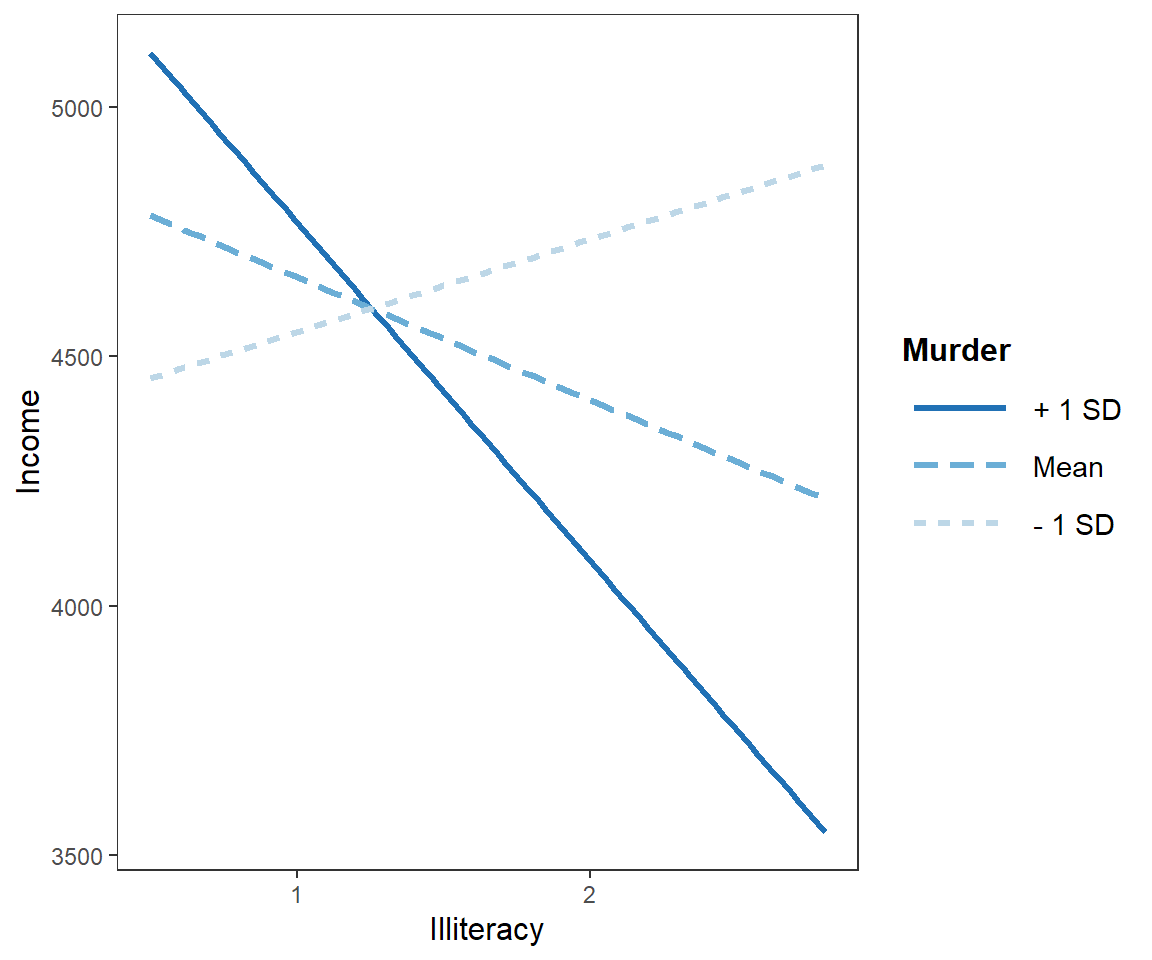Keep in mind that the default behavior of `interact_plot` is to mean-center all variables not involved in the interaction so that the predicted values are more easily interpreted. You can disable that by adding `centered = "none"`. You can choose specific variables by providing their names in a vector to the `centered` argument.

By default, with a continuous moderator you get three lines — 1 standard deviation above and below the mean and the mean itself. If you specify `modx.values = "plus-minus"`, the mean of the moderator is not plotted, just the two +/- SD lines. You may also choose `"terciles"` to split the data into three equal-sized groups — representing the upper, middle, and lower thirds of the distribution of the moderator — and get the line that represents the median of the moderator within each of those groups.

If your moderator is a factor, each level will be plotted and you should leave `modx.values = NULL`, the default.

``````fitiris <- lm(Petal.Length ~ Petal.Width * Species, data = iris)
interact_plot(fitiris, pred = "Petal.Width", modx = "Species")``````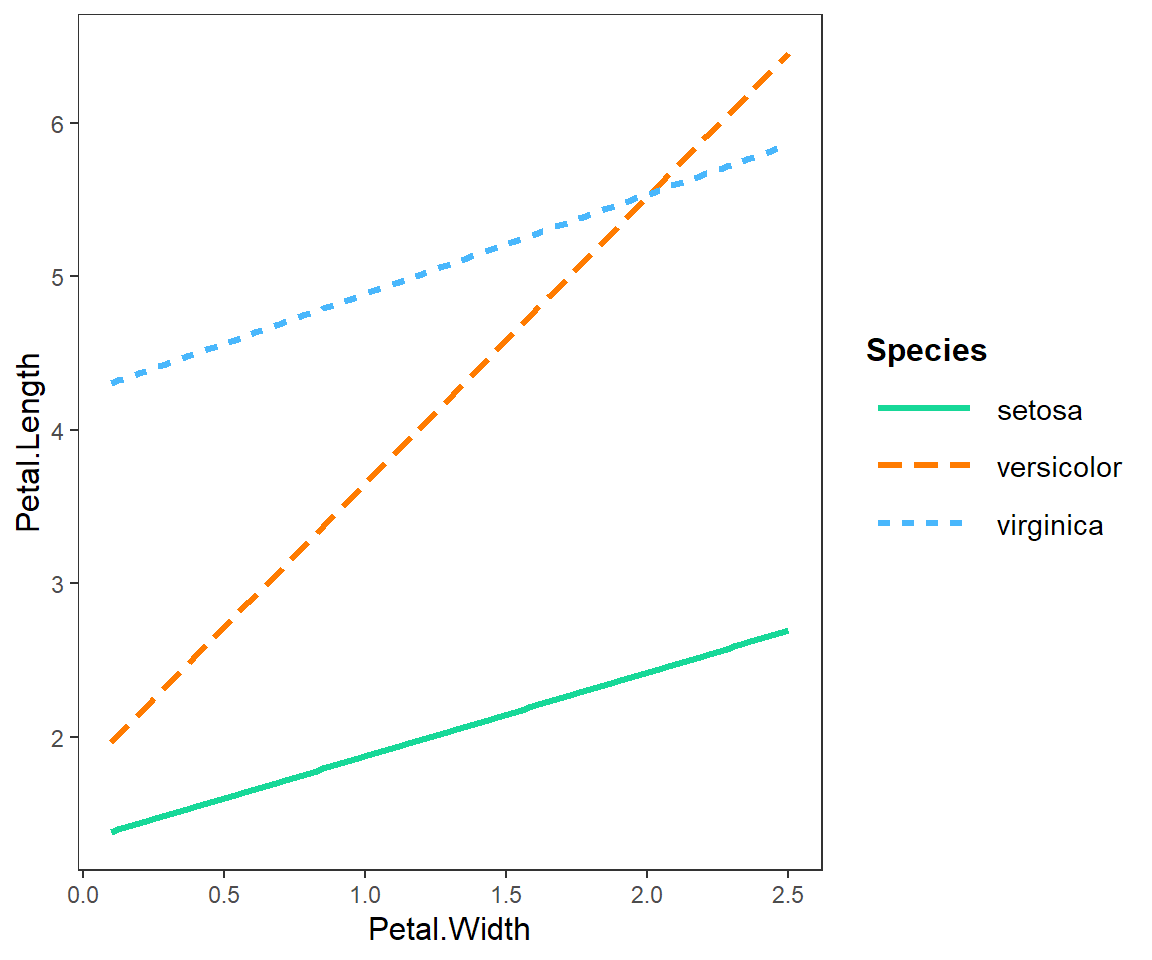If you want, you can specify a subset of a factor’s levels via the `modx.values` argument:

``````interact_plot(fitiris, pred = "Petal.Width", modx = "Species",
modx.values = c("versicolor", "virginica"))``````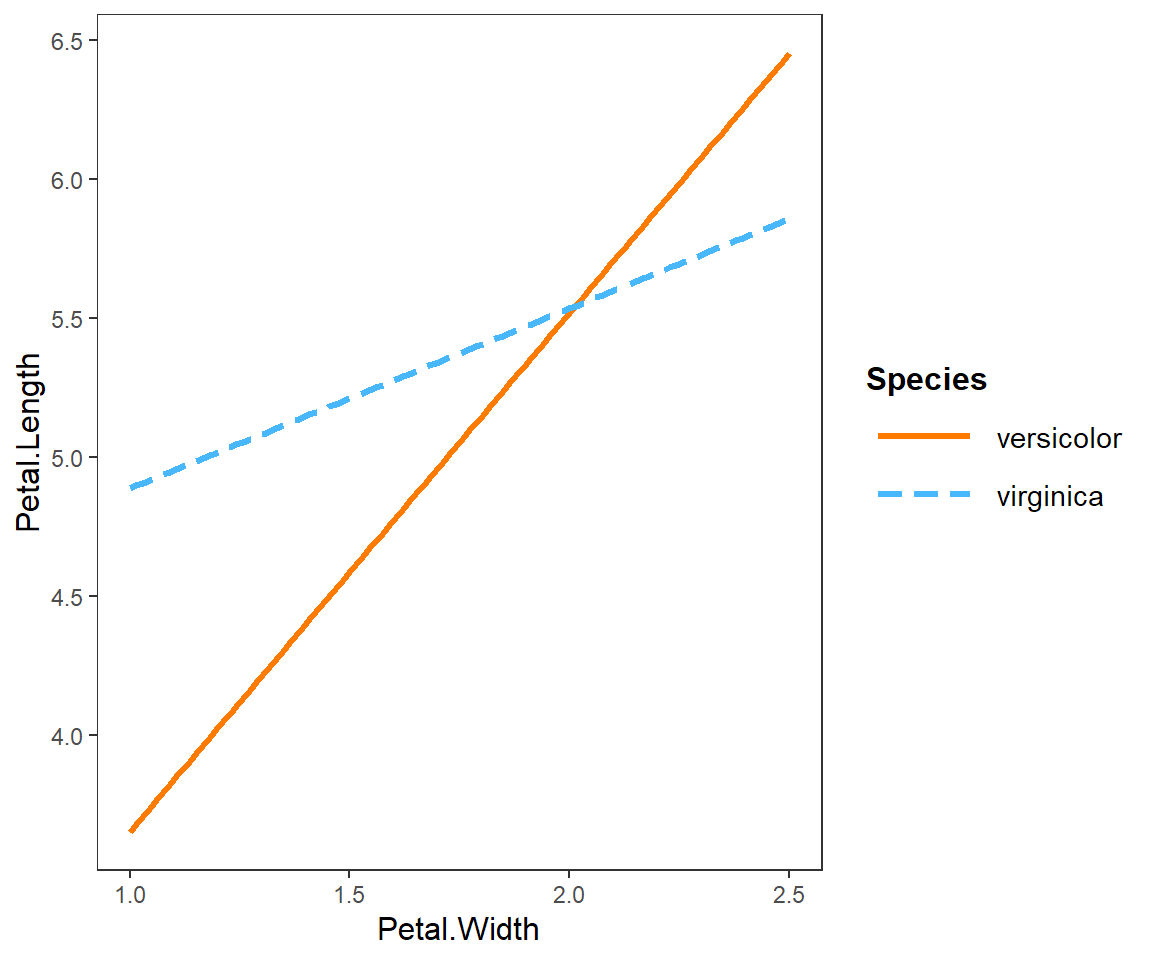## Plotting observed data

If you want to see the individual data points plotted to better understand how the fitted lines related to the observed data, you can use the `plot.points = TRUE` argument.

``interact_plot(fiti, pred = "Illiteracy", modx = "Murder", plot.points = TRUE)``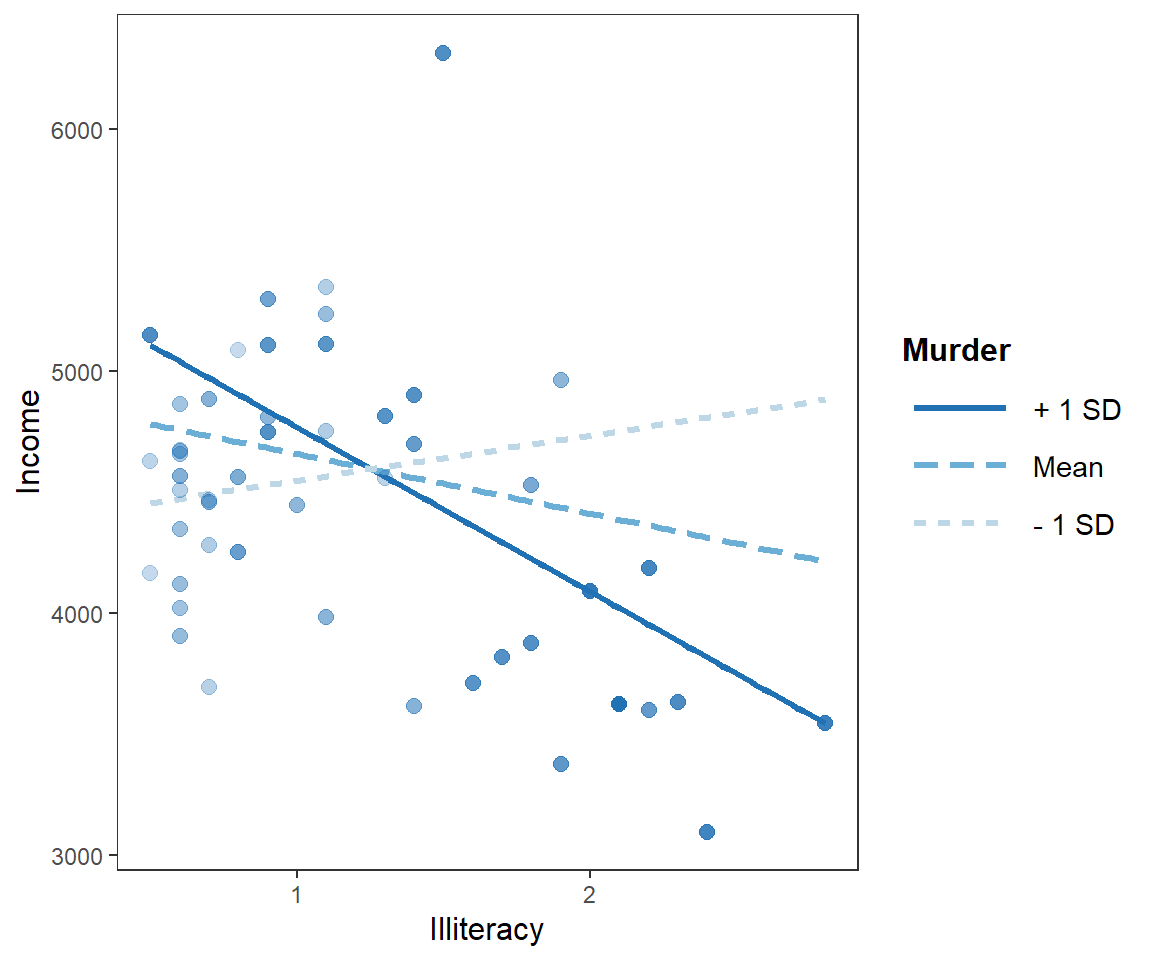For continuous moderators, as you can see, the observed data points are shaded depending on the level of the mdoerator variable.

It can be very enlightening, too, for categorical moderators.

``````interact_plot(fitiris, pred = "Petal.Width", modx = "Species",
plot.points = TRUE)``````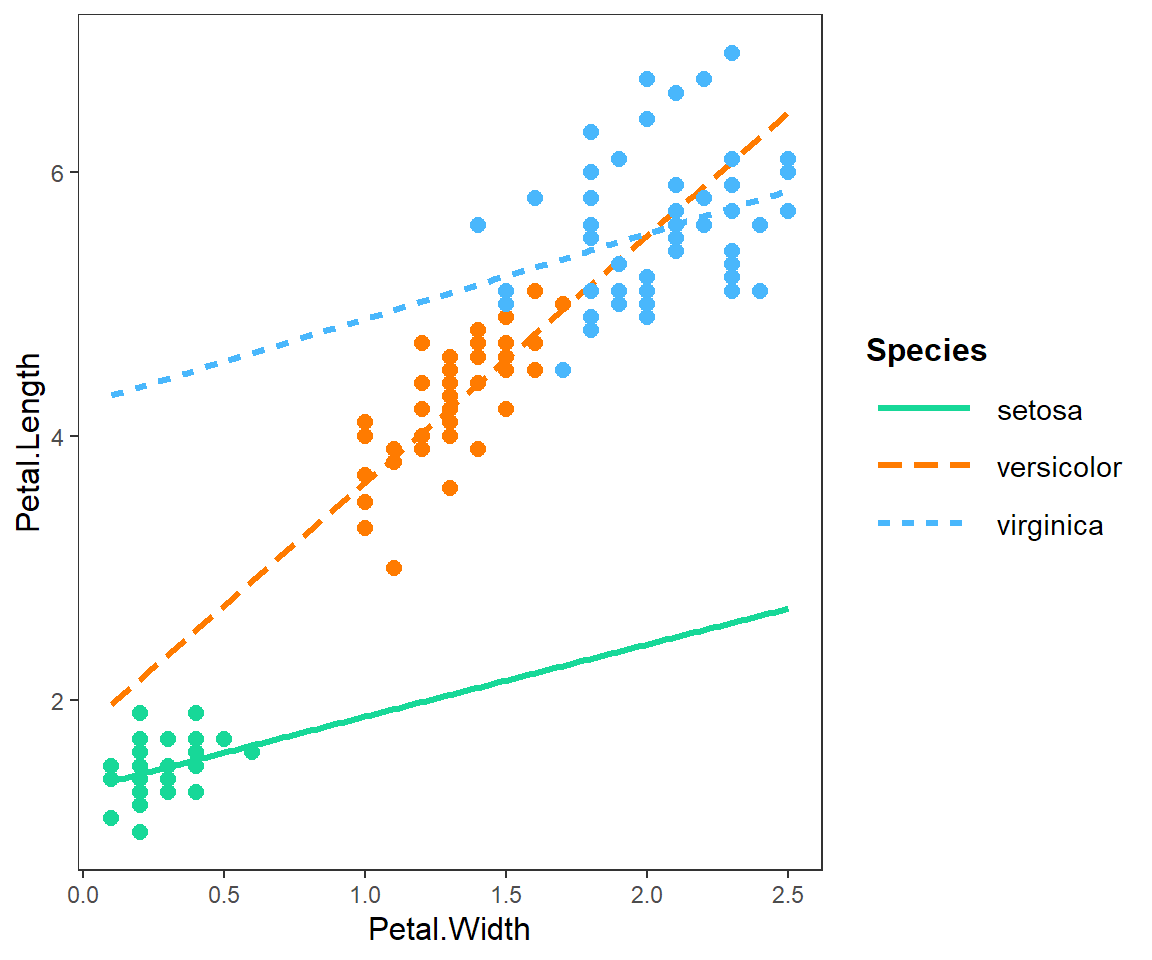Where many points are slightly overlapping as they do here, it can be useful to apply a random “jitter” to move them slightly to stop the overlap. Use the `jitter` argument to do this. If you provide a single number it will apply a jitter of proportional magnitude both sideways and up and down. If you provide a vector length 2, then the first is assumed to refer to the left/right jitter and the second to the up/down jitter.

If you would like to better differentiate the points with factor moderators, you can use `point.shape = TRUE` to give a different shape to each point. This can be especially helpful for black and white publications.

``````interact_plot(fitiris, pred = "Petal.Width", modx = "Species",
plot.points = TRUE, jitter = 0.1, point.shape = TRUE)``````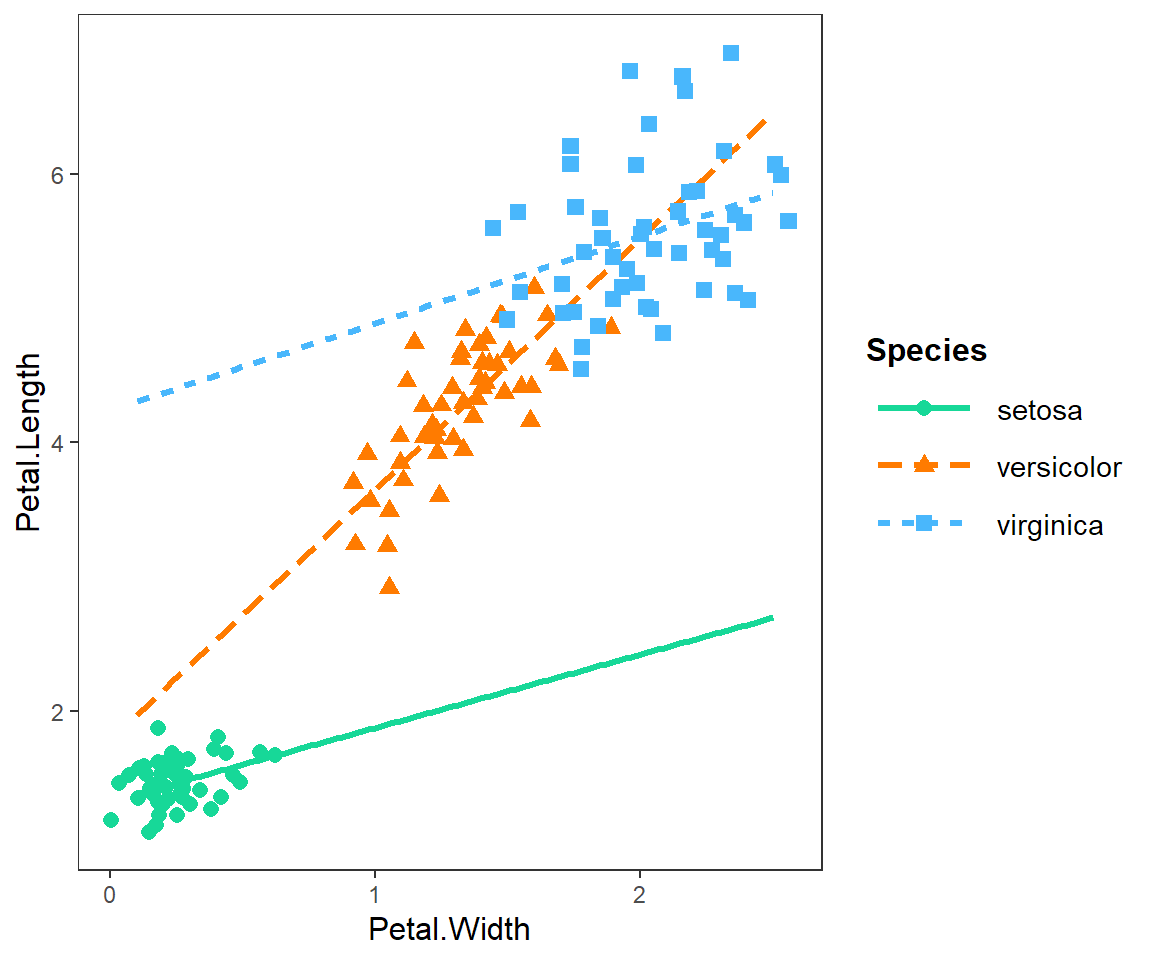If your original data are weighted, then the points will be sized based on the weight. For the purposes of our example, we’ll weight the same model we’ve been using with the population of each state.

``````fiti <- lm(Income ~ Illiteracy * Murder, data = states,
weights = Population)
interact_plot(fiti, pred = "Illiteracy", modx = "Murder", plot.points = TRUE)``````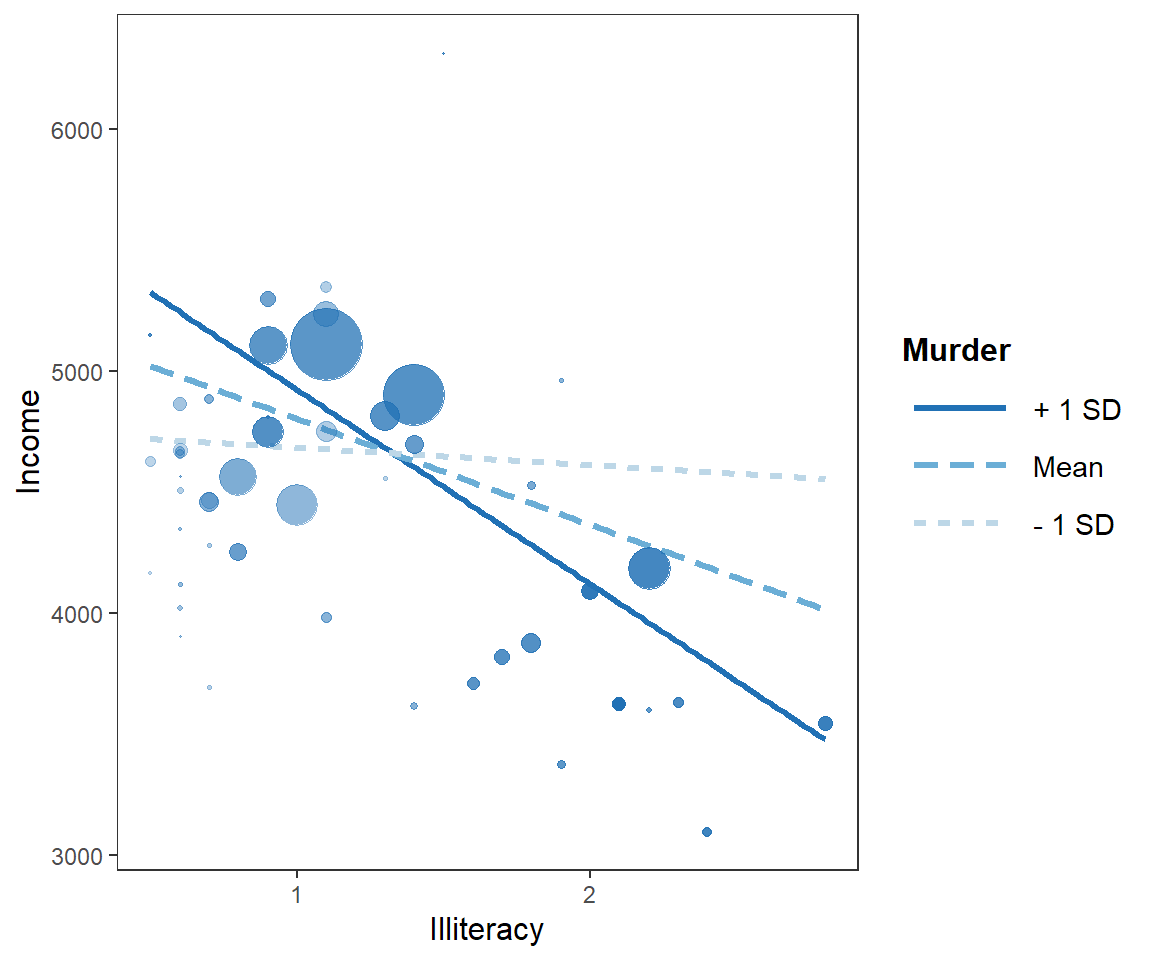For those working with weighted data, it can be hard to use a scatterplot to explore the data unless there is some way to account for the weights. Using size is a nice middle ground.

## Confidence bands

Another way to get a sense of the precision of the estimates is by plotting confidence bands. To get started, just set `interval = TRUE`. To decide how wide the confidence interval should be, express the percentile as a number, e.g., `int.width = 0.8` corresponds to an 80% interval.

``````interact_plot(fiti, pred = "Illiteracy", modx = "Murder", interval = TRUE,
int.width = 0.8)``````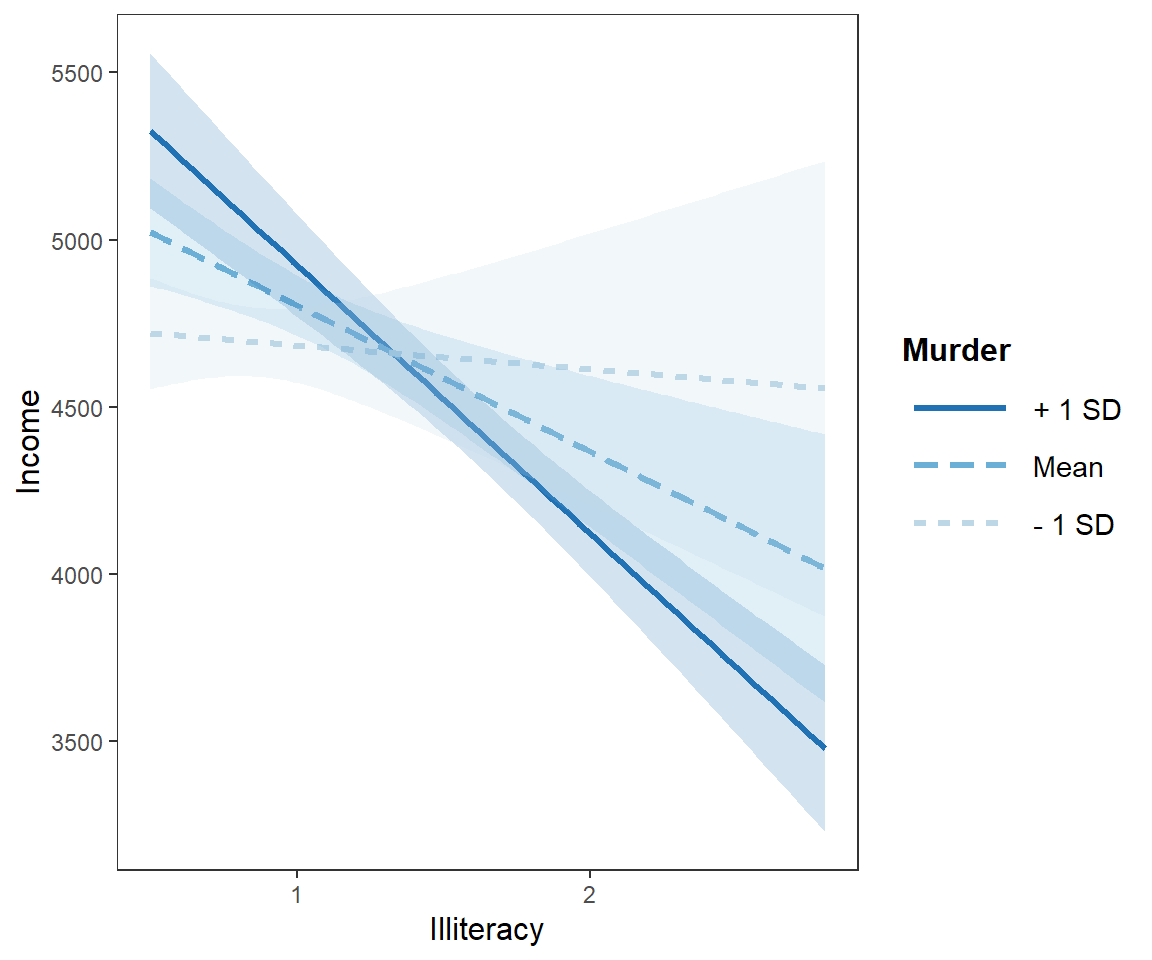You can also use the `robust` argument to plot confidence intervals based on robust standard error calculations.

## Check linearity assumption

A basic assumption of linear regression is that the relationship between the predictors and response variable is linear. When you have an interaction effect, you add the assumption that relationship between your predictor and response is linear regardless of the level of the moderator.

To show a striking example of how this can work, we’ll generate two simple datasets to replicate Hainmueller et al. (2017).

The first has a focal predictor \(X\) that is normally distributed with mean 3 and standard deviation 1. It then has a dichotomous moderator \(W\) that is Bernoulli distributed with mean probability 0.5. We also generate a normally distributed error term with standard deviation 4.

``````set.seed(99)
x <- rnorm(n = 200, mean = 3, sd = 1)
err <- rnorm(n = 200, mean = 0, sd = 4)
w <- rbinom(n = 200, size = 1, prob = 0.5)

y_1 <- 5 - 4*x - 9*w + 3*w*x + err``````

We fit a linear regression model with an interaction between x and w.

``````model_1 <- lm(y_1 ~ x * w)
summ(model_1)``````
 Observations 200 Dependent variable y_1 Type OLS linear regression
 F(3,196) 25.93 R² 0.28 Adj. R² 0.27
Est. S.E. t val. p
(Intercept) 3.19 1.29 2.47 0.01 *
x -3.59 0.42 -8.61 0.00 ***
w -7.77 1.90 -4.10 0.00 ***
x:w 2.86 0.62 4.62 0.00 ***
Standard errors: OLS

In the following plot, we use `linearity.check = TRUE` argument to split the data by the level of the moderator \(W\) and plot predicted lines (black) and a loess line (red) within each group. The predicted lines come from the full data set. The loess line looks only at the subset of data in each pane and will be curved if the relationship is nonlinear. What we’re looking for is whether the red loess line is straight like the predicted line.

``````interact_plot(model_1, pred = "x", modx = "w", linearity.check = TRUE,
plot.points = TRUE)``````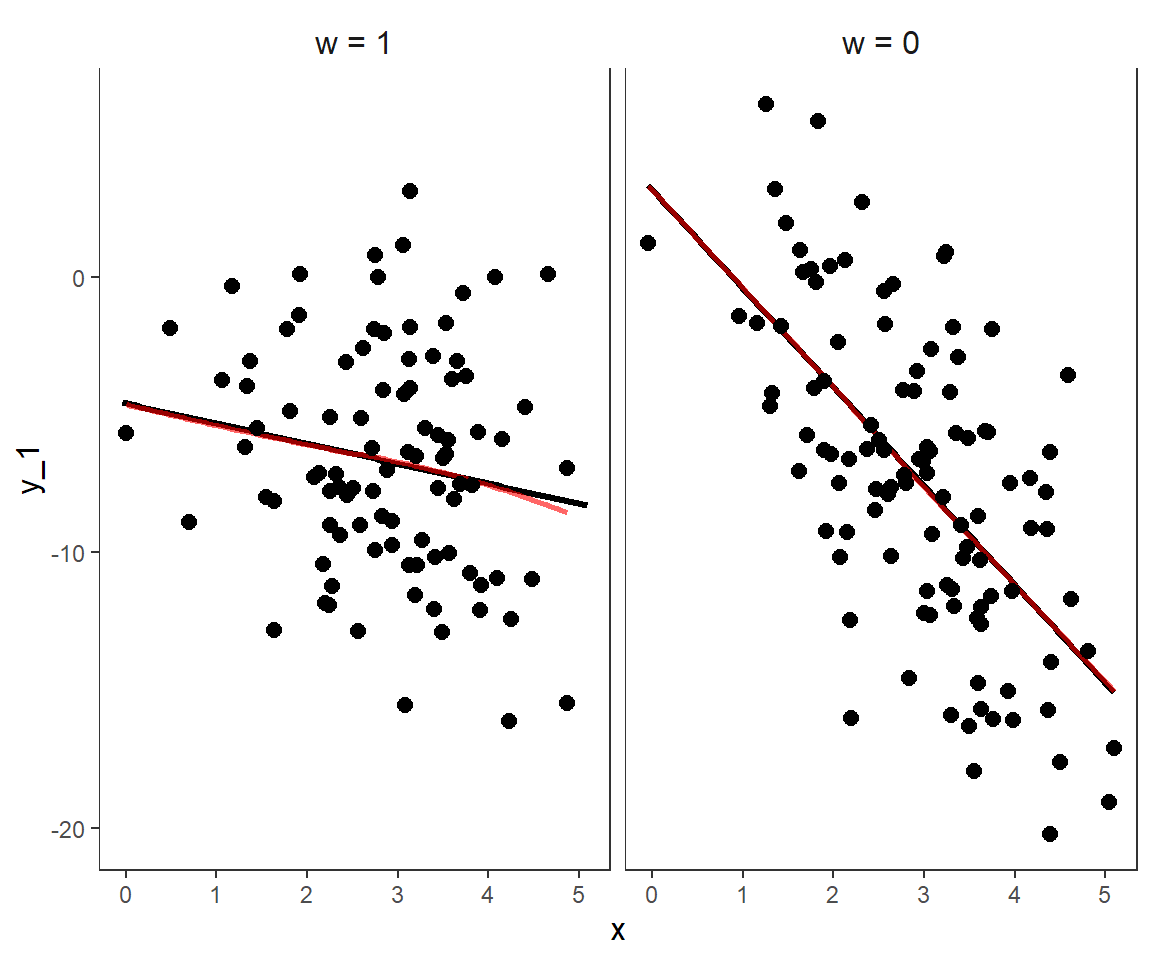In this case, it is. That means the assumption is satisfied.

Now we generate similar data, but this time the linearity assumption will be violated. \(X_2\) will now be uniformly distributed across the range of -3 to 3. The true relationship between `y_2` and \(X_2\) (`x_2`) is non-linear.

``````x_2 <- runif(n = 200, min = -3, max = 3)
y_2 <- 2.5 - x_2^2 - 5*w + 2*w*(x_2^2) + err
data_2 <- as.data.frame(cbind(x_2, y_2, w))

model_2 <- lm(y_2 ~ x_2 * w, data = data_2)
summ(model_2)``````
 Observations 200 Dependent variable y_2 Type OLS linear regression
 F(3,196) 4.26 R² 0.06 Adj. R² 0.05
Est. S.E. t val. p
(Intercept) -1.26 0.48 -2.60 0.01 *
x_2 0.06 0.27 0.20 0.84
w 1.67 0.70 2.38 0.02 *
x_2:w 0.76 0.41 1.84 0.07 .
Standard errors: OLS

The regression output would lead us to believe there is no interaction.

Let’s do the linearity check:

``````interact_plot(model_2, pred = "x_2", modx = "w", linearity.check = TRUE,
plot.points = TRUE)``````